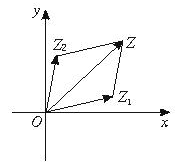# 复数减法的运算

## 复数减法的运算

2017-01-12 作者: xuzhiping 浏览: 3775 次

### 复数的减法运算$$z1 = a + bj$$

$$z2 = c + dj$$

$$z1 + z2 = (a + bj) - (c + dj) = (a - c) + (b - d)j$$